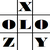anything in Excel...

It's Excel-o-lozy...

### Shortcuts - Row and Column related quick tips...

• Ctrl + "-" (Minus)
It Deletes selected cell/s
Logic - minus means Delete
• Ctrl + Shft + "+" (Plus)
Inserts blank cell/s at selection
Logic - Plus means Add, but remember to press shift
• Ctrl + 9
Hides column/s in selection range
Logic - Key 9 means Key "(" which means C and C for Column
• Ctrl + Shft + 9
Unhides column/s in selection range
Logic - Key 9 means Key "(" which means C and C for Column
• Ctrl + 0 (Zero)
Hides Row/s in selection range
Logic - Key 0 means Key ")", If Key "(" means column then Key ")"means Row
• Ctrl + Shft + 0 (Zero)
Unhides row/s in selection range
Logic - Key 0 means Key ")", If Key "(" means column then Key ")"means Row
• Ctrl + Spacebar
Selects Column/s in selection range
Logic - C for Ctrl, C for Column
• Shift + Spacebar
Selects Row/s in selection range
Logic - Don't ask me logic every time